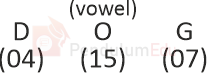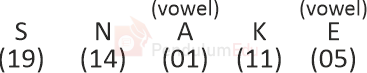Question of The Day06-01-2022

If ‘DOG’ is coded as ‘37’ and ‘CAT’ is coded as ‘47’, Then how will ‘SNAKE’ be coded as?

Correct Answer : a ) 94

Explanation :

According to the question

Let us assume A is numbered as 1, B as 2 and so on till Z being numbered as 26

The common logic applied here is

The Consonants are multiplied by 2 and Vowel are multiplied by 1 and added

Code for DOG is 37⇒ 4 ×2 + 15 × 1 + 7 ×2

⇒ 8 + 15 + 14

⇒ 37

Similarly,

CAT = 47⇒ 3 × 2 + 1 ×1 + 20 ×2

⇒47

Similarly, Code for SNAKE⇒ 19 × 2 + 14 ×2 + 1 × 1 + 11 × 2 + 5 ×1

⇒38 + 28 + 1 + 22 + 5

⇒ 94

Thus, the code for SNAKE is 94

Hence, (a) is the correct answer.0